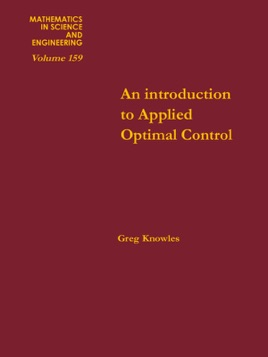• USD 69.99

## Descripción de editorial

Example 1 Consider a mechanism, such as a crane or trolley, of
mass m which moves along a horizontal track without friction. If x( t) represents
the position at time t, we assume the motion of the trolley is
governed by the law
mx(t) = u(t), t > 0, (1)
where u(t) is an external controlling force that we apply to the trolley
(see Fig. 1). Assume that the initial position and velocity of the trolley
are given as x(O) = xo,x(O) = Yo, respectively.